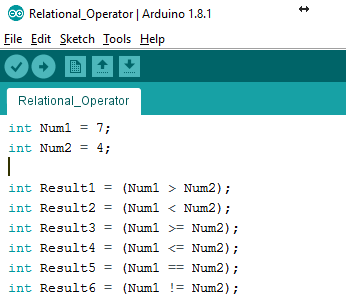Search
•Puriphico

# comparison operators in arduino programming

Learn the basic comparison operators used when programming an Arduino microcontroller.

Definition:

• Comparison operators are mathematical symbols that compare the left variable to the right variable (with respect to the operator, which falls in the middle), and can be evaluated as true or false.

• Comparison operators work best when comparing variables from the same data type; otherwise, they could possibly yield unpredictable results.

• Below find concrete examples of comparison operators:• != (not equal to)

• This operator would yield true if the two variables compared are unequal.

• < (less than)

• <= (less than or equal to)

• == (equal to)

• It is very important to use two equal signs - this is not a typo!

• One equal sign is used to specify the value of a constant, whereas two are used in comparison operations.

• > (greater than)

• >= (greater than or equal to)

Here is an example of a comparison operator from my handwashing program: